Related Articles

# Python | Pandas dataframe.infer_objects()

• Last Updated : 19 Nov, 2018

Python is a great language for doing data analysis, primarily because of the fantastic ecosystem of data-centric python packages. Pandas is one of those packages and makes importing and analyzing data much easier.

Pandas` dataframe.infer_objects()` function attempts to infer better data type for input object column. This function attempts soft conversion of object-dtyped columns, leaving non-object and unconvertible columns unchanged. The inference rules are the same as during normal Series/DataFrame construction.

Syntax: DataFrame.infer_objects()
Returns : converted : same type as input object

Example #1: Use `infer_objects()` function to infer better data type.

 `# importing pandas as pd``import` `pandas as pd`` ` `# Creating the dataframe ``df ``=` `pd.DataFrame({``"A"``:[``"sofia"``, ``5``, ``8``, ``11``, ``100``],``                   ``"B"``:[``2``, ``8``, ``77``, ``4``, ``11``],``                   ``"C"``:[``"amy"``, ``11``, ``4``, ``6``, ``9``]})`` ` `# Print the dataframe``df`

Output :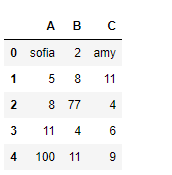Let’s see the dtype (data type) of each column in the dataframe.

 `# to print the basic info``df.info()`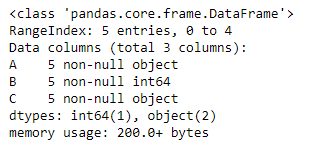As we can see in the output, first and third column is of `object` type. whereas the second column is of `int64` type. Now slice the dataframe and create a new dataframe from it.

 `# slice from the 1st row till end``df_new ``=` `df[``1``:]`` ` `# Let's print the new data frame``df_new`` ` `# Now let's print the data type of the columns``df_new.info()`

Output :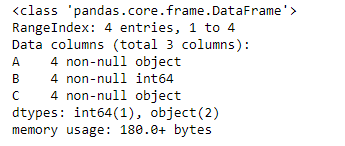As we can see in the output, column “A” and “C” are of object type even though they contain integer value. So, let’s try the `infer_objects()` function.

 `# applying infer_objects() function.``df_new ``=` `df_new.infer_objects()`` ` `# Print the dtype after applying the function``df_new.info()`

Output :Now, if we look at the dtype of each column, we can see that the column “A” and “C” are now of `int64` type.

Example #2: Use `infer_objects()` function to infer better data type for the object.

 `# importing pandas as pd``import` `pandas as pd`` ` `# Creating the dataframe ``df ``=` `pd.DataFrame({``"A"``:[``"sofia"``, ``5``, ``8``, ``11``, ``100``], ``                   ``"B"``:[``2` `+` `2j``, ``8``, ``77``, ``4``, ``11``],``                   ``"C"``:[``"amy"``, ``11``, ``4``, ``6``, ``9``]})`` ` `# Print the dataframe``df`Let’s see the dtype (data type) of each column in the dataframe.

 `# to print the basic info``df.info()`As we can see in the output, first and third column is of `object` type. whereas the second column is of `complex128` type. Now slice the dataframe and create a new dataframe from it.

 `# slice from the 1st row till end``df_new ``=` `df[``1``:]`` ` `# Let's print the new data frame``df_new`` ` `# Now let's print the data type of the columns``df_new.info()`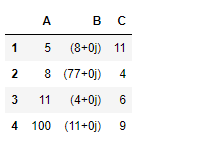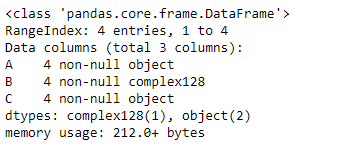As we can see in the output, column “A” and “C” are of object type even though they contain integer value. Similar is the case with column “B”. So, let’s try the `infer_objects()` function.

 `# applying infer_objects() function.``df_new ``=` `df_new.infer_objects()`` ` `# Print the dtype after applying the function``df_new.info()`

Output :Notice, the dtype for column “B” did not change. `infer_objects()` function tries to do soft conversion leaving non-object and unconvertible columns unchanged.

Attention geek! Strengthen your foundations with the Python Programming Foundation Course and learn the basics.

To begin with, your interview preparations Enhance your Data Structures concepts with the Python DS Course. And to begin with your Machine Learning Journey, join the Machine Learning – Basic Level Course

My Personal Notes arrow_drop_up